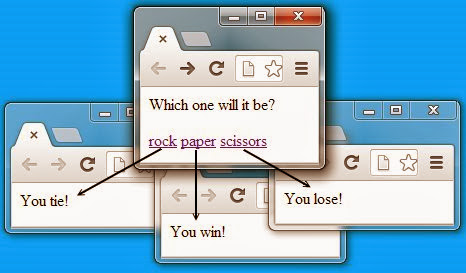# A simple tutorial on using the JavaScript event model

## To be great at JavaScript languages like JQuery, Dojo and YUI, you must master the basics of JavaScript. Here we look at implementing a simple Roshambo application using the JavaScript event model.

This tutorial on using the JavaScript event model picks up from the previous tutorial that introduced a little bit of JavaScripting and DOM traversals.

Our Rashambo application has three links, one for rock, paper and scissors:

```

<p>Which one will it be?</p>
<div id="choices">
<a id="rock" href="#">rock</a>
<a id="paper" href="#">paper</a>
<a id="scissors" href="#">scissors</a>
</div>
```

These links look like they lead nowhere, but nothing could be further from the truth. By traversing the DOM, we make each of these links call the popUpResult JavaScript method. This DOM traversal code was introduced in the previous example, although a quick change is made here to have the onclick event of an anchor link call the popUpResult method:

```

var element = document.getElementById('choices');
var anchors   = element.getElementsByTagName('a');
for (var i=0; i < anchors.length; i++ ) {
anchors[i].onclick = popUpResult;
}
}
```

This iteration of the Roshambo application focuses on events. This is seen both by the fact that the anchor links are having their onclick event associated with the popUpResult method, along with the fact that the popUpResult method is being reprogrammed to interact with the JavaScript event model.

First, you will notice that the popUpResult method is passed an event object named evt. Secondly, from this evt variable we can find out which element fired off the event, and what the id attribute was for that element. This information can tell us whether the end user click on the rock, paper or scissors link.

```

popUpResult = function(evt){
var src = evt.srcElement;
var id = src.getAttribute("id");
var result;

if (id=='rock') {
result = "tie";
}
if (id=='paper') {
result = "win";
}
if (id=='scissors') {
result = "lose";
}
newWindow = open("","","top=200,left=200,height=200,width=400");
newWindow.document.write("You " + result + "!");
}
```

A little conditional logic is performed to figure out if the game play ended up in a win, loss or tie for the end user. An nested else block would have been more efficient to use here, but the three if statements make the code a bit easier to read.

Finally, the JavaScript code pops up a new window. These windows do not display existing HTML pages as the previous iteration did, but instead, create blank windows to which the result is written. So not only does this iteration use the JavaScript event model, but it also eliminates the need for three superfluous files: win.html, lose.html and tie.html. When the page is run in a browser, the result is the same as with the previous iteration. All that has changed is the implementation behind the scenes.Here is the complete code for this example.

```<html>

<script>

var element = document.getElementById('choices');
var anchors   = element.getElementsByTagName('a');
for (var i=0; i < anchors.length; i++ ) {
anchors[i].onclick = popUpResult;
}
}

popUpResult = function(evt){
var src = evt.srcElement;
var id = src.getAttribute("id");
var result;

if (id=='rock') {
result = "tie";
}
if (id=='paper') {
result = "win";
}
if (id=='scissors') {
result = "lose";
}
newWindow = open("","","top=200,left=200,height=200,width=400");
newWindow.document.write("You " + result + "!");
}

</script>

<body>
<p>Which one will it be?</p>
<div id="choices">
<a id="rock" href="#">rock</a>
<a id="paper" href="#">paper</a>
<a id="scissors" href="#">scissors</a>
</div>
</body>
</html>
```

Close